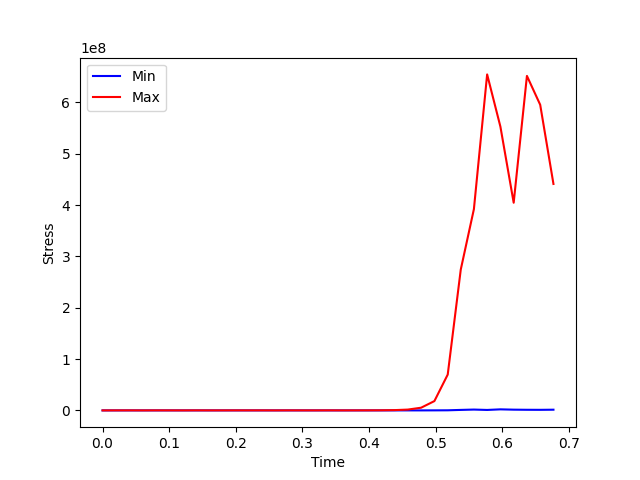# Use incremental evaluation helper#

This example shows you how to use the incremental evaluation helper.

```# Import necessary modules
from ansys.dpf import core as dpf
from ansys.dpf.core import examples
```

Retrieve an example to instantiate a DataSources object

```path = examples.download_transient_result()
ds = dpf.DataSources(path)

# From the DataSources object we can retrieve the scoping
# In this example we want to compute the min/max for all the time sets
tf_support = tf_provider.get_output(output_type=dpf.types.time_freq_support)
scoping = dpf.time_freq_scoping_factory.scoping_on_all_time_freqs(tf_support)

# If you don't need to reuse TimeFreqSupport you could also use the DataSources
# scoping = dpf.time_freq_scoping_factory.scoping_on_all_time_freqs(ds)
```

Defining the workflow to exploit

```# Instantiating a streams_provider is important when dealing with incremental evaluation
# due to multiple reuses of operators

# Defining the main workflow
result_op = dpf.operators.result.stress(
data_sources=ds, time_scoping=scoping, streams_container=streams_provider
)
norm_fc = dpf.operators.math.norm_fc(result_op)
final_op = dpf.operators.min_max.min_max_fc_inc(norm_fc)
```

Obtain a new operator to retrieve outputs from

```# Workflow is adapted from the first and the last operator in the current workflow
# Scoping is important to split the workload into chunks
new_end_op = dpf.split_workflow_in_chunks(result_op, final_op, scoping)

# Obtain results on the same pin numbers
min = new_end_op.get_output(0, dpf.types.field)
max = new_end_op.get_output(1, dpf.types.field)

# Plot results
import matplotlib.pyplot as plt

x = tf_support.time_frequencies.data
plt.plot(x, min.data, "b", label="Min")
plt.plot(x, max.data, "r", label="Max")
plt.xlabel("Time")
plt.ylabel("Stress")
plt.legend()
plt.show()
``````Estimating chunk_size with max_bytes: 1073741824
Done. chunk_size set to 35 (scoping size: 35)
```

Total running time of the script: ( 0 minutes 3.606 seconds)

Gallery generated by Sphinx-Gallery